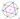## 钝角三角形的概率

Thu, 30th January 2020Edit on Github概率三角形正方形

54张牌,地主里面，地主得到20张牌，那么地主得到炸弹的概率是多少（得到4个一样或者对王）?

wayne快速给出了答案，然后他们引申出了平面图形中随机选择三个点，构成钝角三角形的一系列概率问题.

# 扑克牌问题

1）计算54张牌分成17,17,20三堆，且不存在4条和对王的所有情况：

Select[CoefficientRules[((x+y+z)^4-x^4-y^4-z^4)^13 ((x+y+z)^2-x^2-y^2-z^2 ),{x,y,z}],Min[#[]]==17&&Max[#[]]==20&]

2）计算54张牌分成17,17,20三堆的所有可能情况：Multinomial[17, 17, 20] =749991557119474188218700
3）有炸弹的概率就是：
$1-\frac{341495369180333055148032}{749991557119474188218700}=\frac{79350463857642022741}{145686005656463517525} \approx 0.5446677153$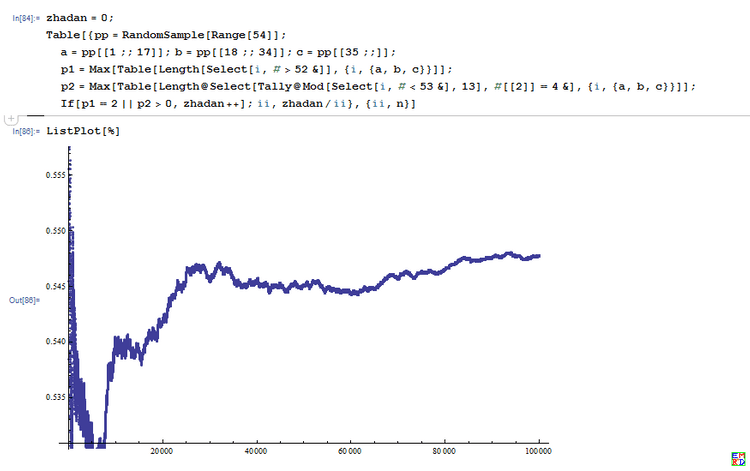$((x+1)^4-x^4)^13 ((x+1)^2 -x^2)$$x^{20}$的系数 223786474655744 除以 $C_{54}^{20}$.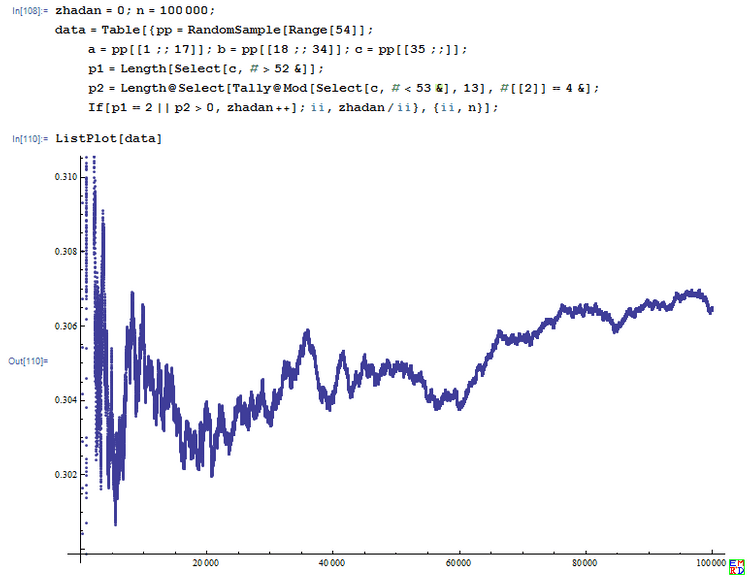# 钝角三角形概率问题

## 圆周问题

sheng_jianguo给出如下的解答:

hujunhua给出了对应的二维情况的图解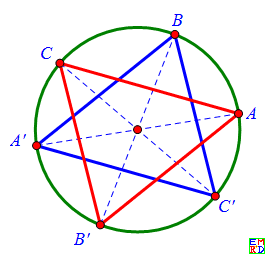$\pi\lt\alpha\lt0$时（红标记角）, 点B同样位于OA弧的对顶角弧上时才是锐角三角形，这时$\pi+\alpha\lt\beta\lt\pi$，对应于下图2左上角的灰色三角区。在其它位置都是钝角三角形，对应于下图2中的浅绿色区。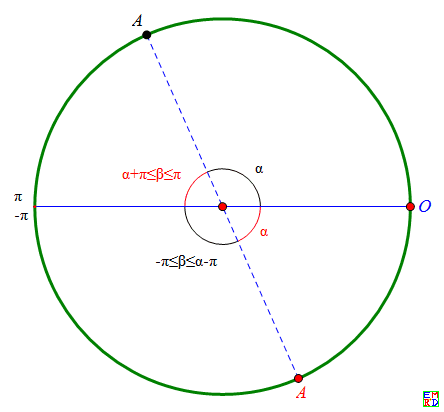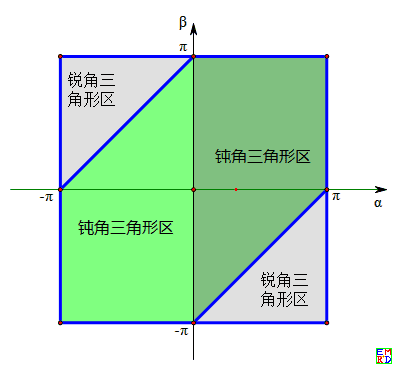kastin基于三个点的不同分布概率，使用积分模型也给出了很多不同的结论
mathe给出了一种通过积分计算的方案:

$F_3(t)=\int_{s=0}^t(4t-2s)ds=3t^2$,
$F_4(t)=\int_{s=0}^t(2t-s)\times 6sds=4t^3$.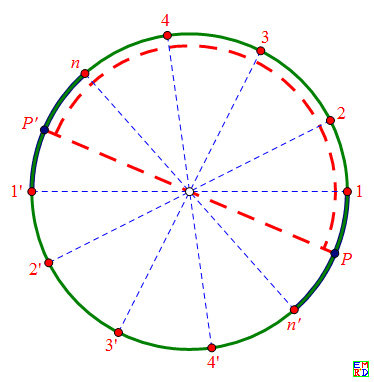sheng_jianguo进一步将问题推广到高维

## 正方形情况

wayne发现百度贴吧里面已经有人通过复杂的符号计算得出了结果$\frac{97}{150}+\frac{\pi}{40}\approx0.72520648300641149763$并且这个结果和他的数值模拟结果非常匹配。
Lwins_G给出:

1. 一个角是否为钝角，可以用形成该角的两条有向边的内积（有向边看作向量）的正负来判别。
2. 一个三角形为钝角三角形，当且仅当其恰好有一个钝角。
故而，这归结为计算
$3 \int_0^1 \int_0^1 \int_0^1 \int_0^1 \int_0^1 \int_0^1 f((x_2 - x_1)(x_3 - x_1) + (y_2 - y_1)(y_3 - y_1)) dx_1 dy_1 dx_2 dy_2 dx_3 dy_3 .$
其中$f(x)$$x \lt 0$时取$1$，否则取$0$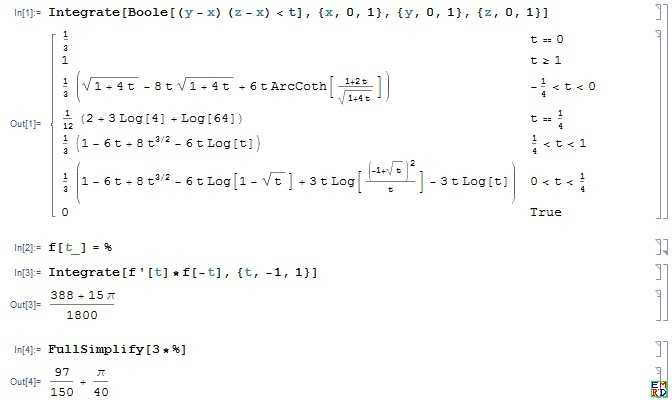从而验证了百度贴吧中结果的正确性。
Github